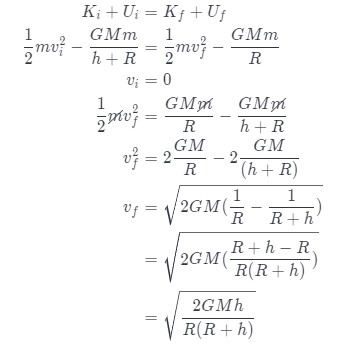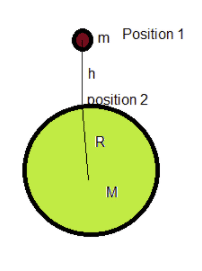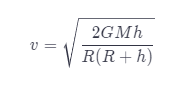# An Object Of Mass M Is Dropped From Height H Above A Planet Of Mass M And Radius R.

We thoroughly check each answer to a question to provide you with the most correct answers. Found a mistake? Let us know about it through the REPORT button at the bottom of the page.

An object of mass m is dropped from height h above a planet of mass M and radius R. Find an expression for the object’s speed as it hits the ground.

Mass of object = m

Height above planet = h

Mass of the planet = M

The object was thrown from the given height, we need to determine its velocity when it hits the surface of the planet. For this we can apply conservation of energy at two points i.e when the object was at given height and the other is when it hit the surface (as shown in figure).

Initial energy = Final energy